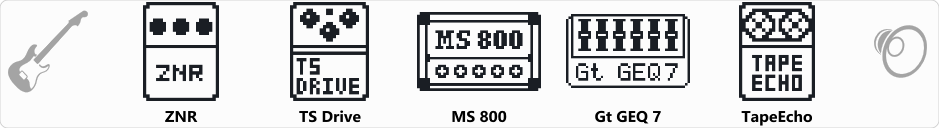# 80s Dokken/Lynch Style Tone

Discussion in 'Zoom G3n/G3Xn/G5n' started by jriff79, Dec 19, 2019.

1. 80s Dokken/Lynch Style ToneDevice: Zoom G3n
Firmware: 2.20

Name on device: Dokken
Optimized for: Guitar Amp

Effects chain:This massive drive sound combines TS Drive and MS 800. Best if used with real 4x12 cabinet or modeling amp with amp turned off and only the cabinet model turned on such as a Line 6 Spider V.

Effect: "ZNR" (Dynamics), active - "yes"
"Detect" = EFXIN
"Depth" = 100
"Threshold" = 30
"Decay" = 0

Effect: "TS Drive" (Overdrive / Distortion), active - "yes"
"Gain" = 81
"Boost" = Off
"Tone" = 57
"Volume" = 82

Effect: "MS 800" (Amp simulator), active - "yes"
"Input" = HI
"Bass" = 60
"Middle" = 60
"Treble" = 100
"Presence" = 66
"Gain" = 100
"Volume" = 52
"SOLO" = 3

Effect: "Gt GEQ 7" (Filter), active - "yes"
"100 Hz" = 0.0
"200 Hz" = 0.0
"400 Hz" = 0.0
"800 Hz" = 0.0
"1.6 kHz" = 0.0
"3.2 kHz" = 12.0
"6.4 kHz" = 0.0
"Volume" = 80
"100 Hz" = 0.0
"200 Hz" = 0.0
"400 Hz" = 0.0
"800 Hz" = 0.0
"1.6 kHz" = 0.0
"3.2 kHz" = 0.0
"6.4 kHz" = 0.0
"Volume" = 80
"Parameter set" = A

Effect: "TapeEcho" (Delay), active - "yes"
"Time" = 540
"F.B." = 65
"Mix" = 36
"Tail" = Off

Patch Volume: 15